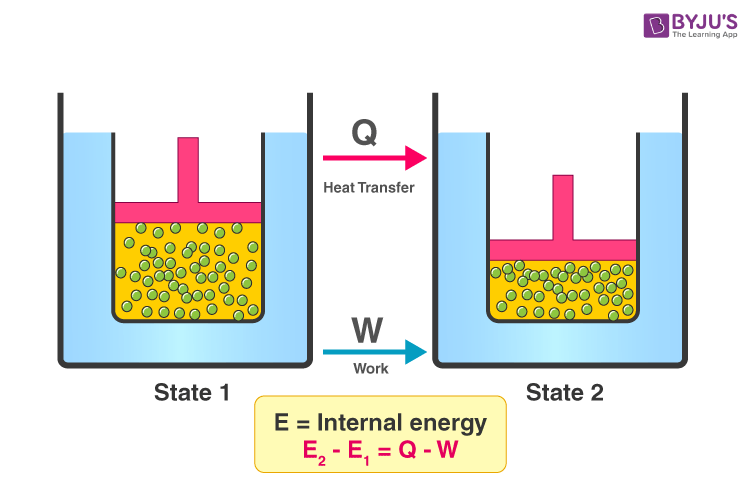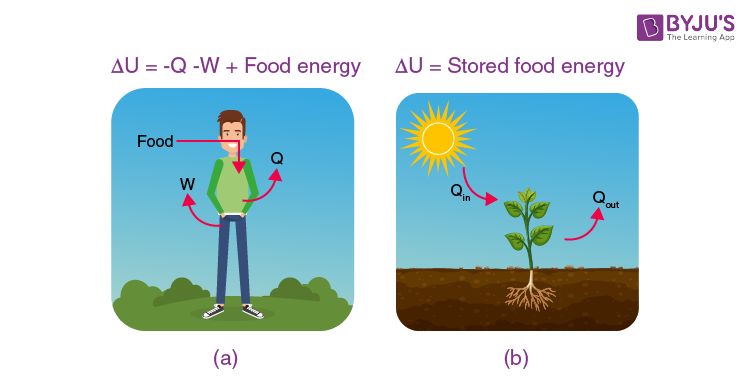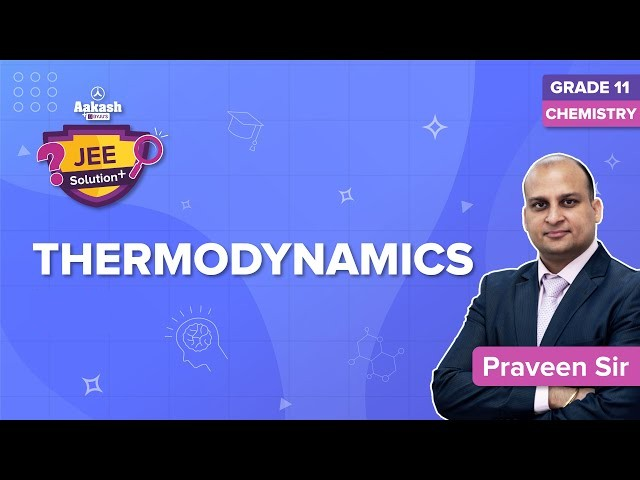Win up to 100% scholarship on Aakash BYJU'S JEE/NEET courses with ABNAT Win up to 100% scholarship on Aakash BYJU'S JEE/NEET courses with ABNAT

# First Law of Thermodynamics

Before we get into the first law of thermodynamics, we need to understand the relation between heat and work and the concept of internal energy. Just like mass, energy is always conserved, i.e., it can neither be created nor destroyed, but it can be transformed from one form to another. Internal energy is a thermodynamic property of the system that refers to the energy associated with the molecules of the system, which includes kinetic energy and potential energy.

Whenever a system goes through any change due to the interaction of heat, work and internal energy, it is followed by numerous energy transfers and conversions. However, during these transfers, there is no net change in the total energy.

Similarly, if we look at the first law of thermodynamics, it affirms that heat is a form of energy. What it means is that the thermodynamic processes are governed by the principle of conservation of energy. The first law of thermodynamics is also sometimes referred to as the law of conservation of energy.

## What Is the First Law of Thermodynamics?A thermodynamic system in an equilibrium state possesses a state variable known as internal energy(E). Between the two systems, the change in the internal energy is equal to the difference of the heat transfer into the system and the work done by the system.

The first law of thermodynamics states that the energy of the universe remains the same. Though it may be exchanged between the system and the surroundings, it can’t be created or destroyed. The law basically relates to the changes in energy states due to work and heat transfer. It redefines the conservation of energy concept.

 First Law of Thermodynamics The first law of thermodynamics states that heat is a form of energy, and thermodynamic processes are, therefore, subject to the principle of conservation of energy. This means that heat energy cannot be created or destroyed. It can, however, be transferred from one location to another and converted to and from other forms of energy.

To help you understand the meaning of the first law, we can take the common example of a heat engine. In a heat engine, the thermal energy is converted into mechanical energy, and the process also is vice versa. Heat engines are mostly categorised as open systems. The basic working principle of a heat engine is that it makes use of the different relationships between heat, pressure and volume of a working fluid which is usually a gas. Sometimes phase changes might also occur involving a gas to liquid and back to gas.

## First Law of Thermodynamics Equation

The equation for the first law of thermodynamics is given as

ΔU = q + W

Where,

• ΔU = Change in internal energy of the system
• q = Algebraic sum of heat transfer between system and surroundings
• W = Work interaction of the system with its surroundings### Points to Remember

• For an isolated system, energy (E) always remains constant.
• Internal energy is a point function and property of the system. Internal energy is an extensive property (mass-dependent), while specific energy is an intensive property (independent of mass).
• For an ideal gas, the internal energy is a function of temperature only.

### Some Examples:

Q. A gas has constant pressure in a system. There is a loss of 45 J of heat in the surroundings around the system. 450 J of work is done on the system. Find the system’s internal energy.

Solution:

ΔU = q+w

ΔU = 45J + 450J

ΔU = 495J

In general, if we want to find the internal energy, which is denoted by ΔU, it is important to consider the relationship between the surroundings and the system. We already know, according to the law, that energy is neither created nor destroyed. Thus, we can tell that anything that is lost by the surroundings will be gained by the system. Moreover, the surrounding area will lose heat and carry out some work on the system. So if we look at q and w, they are positive in the equation, and this is mainly due to the system gaining some heat and work being done on itself.

## First Law of Thermodynamics Limitations

Here, we will discuss the limitations of the first law of thermodynamics. The law states that whenever a system undergoes any thermodynamic process, it always holds a certain energy balance. However, the first law fails to give the feasibility of the process or change of state that the system undergoes.

Also Read: Second Law of Thermodynamics

For instance, the first law fails to explain why heat flows from the hot end to the cold end when a metallic rod is heated at one end and not on the other and vice-versa. The first law only quantifies the energy transfer that takes place during this process. It is the second law of thermodynamics which provides the criterion for the feasibility of various processes. We will take an example and understand the concept further.

### Perpetual Motion Machine of First Kind (PMM1)

It is impossible to construct a machine that can continuously supply mechanical work without consuming any energy simultaneously. Such a hypothetical machine is known as the perpetual motion machine of the first kind. These types of machines violate the 1st law of thermodynamics and do not exist in reality.

## First law of Thermodynamics for a Closed System

Work done for a closed system is the product of pressure applied and the change in volume that occurs due to applied pressure.

w = − P ΔV

Where P is the constant external pressure on the system, and ΔV is the change in the volume of the system. This is specifically called “pressure-volume” work.

The internal energy of a system increases or decreases depending on work interaction that takes place across its boundaries. The internal energy would increase if work is done on the system and decreases if work is done by the system. Any heat interaction that takes place in the system with its surroundings also changes its internal energy. But since energy remains constant (from the first law of thermodynamics), the total change in internal energy is always zero. If energy is lost by the system, then it is absorbed by the surroundings. If energy is absorbed into a system, then it implies that the energy was released by the surroundings.

ΔUsystem = −ΔUsurroundings

Where ΔUsystem is the change in the total internal energy of the system, and ΔUsurroundings is the change in the total energy of the surrounding.

Also Read: Third Law of Thermodynamics

Carefully study the table given below.

 Process Sign Convention for Heat(q) Sign Convention for Work(w) Work done by the system N/A – Work done on the system N/A + Heat extracted from the system – N/A Heat added to the system + N/A

For a closed system

 Process Internal Energy Change Heat (q) Work(w) Example Adiabatic (q=0) +/- 0 +/- An isolated system in which heat neither enters nor leaves. Constant volume(ΔV) (isochoric) +/- +/- 0 A hard, pressure-isolated system like a bomb calorimeter. Constant pressure (isobaric) +/ – Enthalpy − p ΔV Most processes occur in constant external pressure. Isothermal 0 +/- -/+ There is no change of temperature like a temperature bath.

## Video Lessons

### Thermodynamics Important Topics### Thermodynamics Important Questions### Thermodynamics Top 7 Questions### Thermodynamics JEE Solutions### Thermodynamics JEE Main Important Questions### Thermodynamics – Full Chapter Revision### Thermodynamics – Top 15 Most Important and Expected JEE Main Questions## Frequently Asked Questions on First Law of Thermodynamics

Q1

### What are the limitations of the first law of thermodynamics?

It does not tell us about the direction of the flow of heat.
It fails to explain why heat cannot be spontaneously converted into work.

Q2

### What is the mathematical form of the first law of thermodynamics?

dQ = dV+dW
But dW = PdV
Therefore, dQ = dV+PdV
dQ is the heat absorbed by the system.
dV is the internal energy of the system.
dW is the work done by the system.
P is the pressure.
dV is the change in volume.

Q3

### State the first law of thermodynamics.

The law states that the energy entering the system in the form of heat is equal to the sum of the increase in the system’s internal energy and the energy leaving the system in the form of work done by the system on its surroundings.

Q4

### What are the applications of the first law of thermodynamics?

Isobaric process
Isochoric process

Q5

### Is the first law of thermodynamics the law of conservation of mass or energy?

The first law of thermodynamics is the law of conservation of energy.

Q6

### Define irreversible process.

A process in which the system cannot be retraced to its original state is called an irreversible process.

Q7

### What is a reversible process?

A process is said to be reversible when the various stages of an operation to which it is subjected can be traversed back in the opposite direction in such a way that the substance passes through exactly the same conditions at every step in the reverse process as in the direct process.

Q8

### What is an isolated system?

A system is said to be an open system if it can exchange both energy and matter with its surroundings.

Test Your Knowledge On First Law Of Thermodynamics!